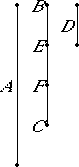# Proposition 4

Any number is either a part or parts of any number, the less of the greater.

Let A and BC be two numbers, and let BC be the less.

I say that BC is either a part, or parts, of A.Either A and BC are relatively prime or they are not.

First, let A and BC be relatively prime.

VII.Def.4

Then, if BC is divided into the units in it, then each unit of those in BC is some part of A, so that BC is parts of A.

Next let A and BC not be relatively prime, then BC either measures, or does not measure, A.

Now if BC measures A, then BC is a part of A. But, if not, take the greatest common measure D of A and BC, and divide BC into the numbers equal to D, namely BE, EF, and FC.

Now, since D measures A, therefore D is a part of A. But D equals each of the numbers BE, EF, and FC, therefore each of the numbers BE, EF, and FC is also a part of A, so that BC is parts of A.

Therefore, any number is either a part or parts of any number, the less of the greater.

Q.E.D.

## Guide

This proposition says that if b is a smaller number than a, then b is either a part of a, that is, b is a unit fraction of a, or b is parts of a, that is, a proper fraction, but not a unit fraction, of a. For instance, 2 is one part of 6, namely, one third part; but 4 is parts of 6, namely, 2 third parts of 6.

It seems obvious that when one number b is less than another a, that since the unit u is a part of a and b is a multiple of u, then b is some multiple of a part of a. Yet, the proof of this proposition ignores that possibility, except in the special case when b and a are relatively prime. In the case of a = 4u and b = 6u, the proof will find that a is 2 third parts of b. Thus, it appears that a satisfactory answer to the question “How mary parts of a is b?” requires finding the least number of parts.

The proof has three cases.

1. If b and a are relatively prime, then b consists of b of the nth parts of a where a = nu.
2. If b divides a, then b is one part of a.
3. Otherwise they’re not relatively prime, and b does not divide a. Let d be their greatest common divisor. Then b is some multiple m of d, that is, b = md, and a is some other multiple n of d, that is, a = nd Therefore, b consists of m one-nth parts of a.

Again, if 1 were considered a number, then the three cases could be consolidated into one.

We'll write the statement such as b is one nth part of a as the equation b = a/n, and a statement such as b consists of m one-nth parts of a as the equation b = (m/n)a.

#### Use of this proposition

This proposition is used in VII.20.